Next: 12.6 Trigonometric Functions Up: 12. Power Series Previous: 12.4 The Exponential Function   Index

# 12.5 Logarithms

12.37   Definition (Logarithm.) Let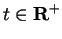. The logarithm of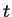is the unique number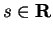such that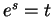. We denote the logarithm ofby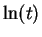, Hence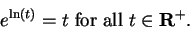(12.38)

12.39   Remark. Since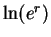is the unique number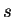such that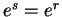, it follows that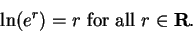(12.40)

12.41   Theorem. For all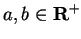,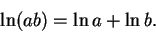Proof:12.42   Exercise. A Show that
a)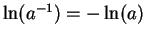for all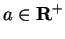.
b)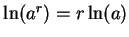for all,.
c)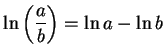for all.

12.43   Remark. It follows from the fact that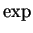is strictly increasing onthat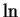is strictly increasing on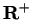: if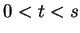, then both of the statements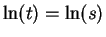and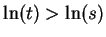lead to contradictions.

12.44   Theorem (Continuity of.)is a continuous function on.

Proof: Let, and let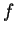be a sequence insuch that. I want to show that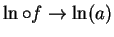. Let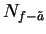be a precision function for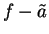. I want to construct a precision functionfor.

Scratchwork: For all, and all,Note that sinceis strictly increasing,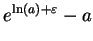and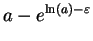are both positive. This calculation motivates the following definition:

For all, let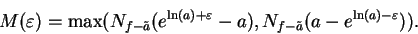Then for all,,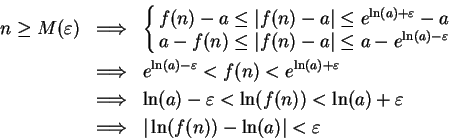Henceis a precision function for.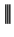12.45   Theorem (Differentiability of.)The functionis differentiable onand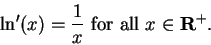Proof: Letand let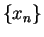be a sequence in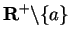. Then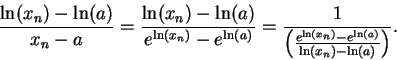(Note, I have not divided by.) Sinceis continuous, I know, and hence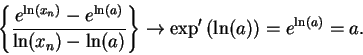Hence,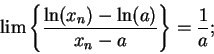i.e.,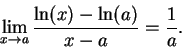This shows that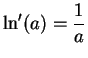.Next: 12.6 Trigonometric Functions Up: 12. Power Series Previous: 12.4 The Exponential Function   Index